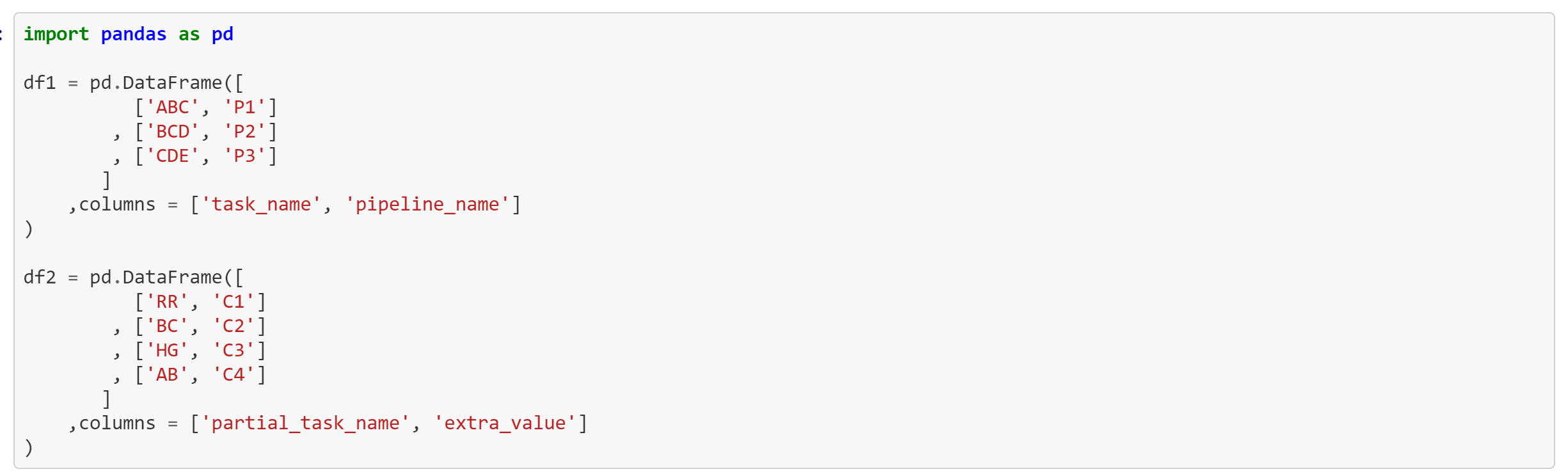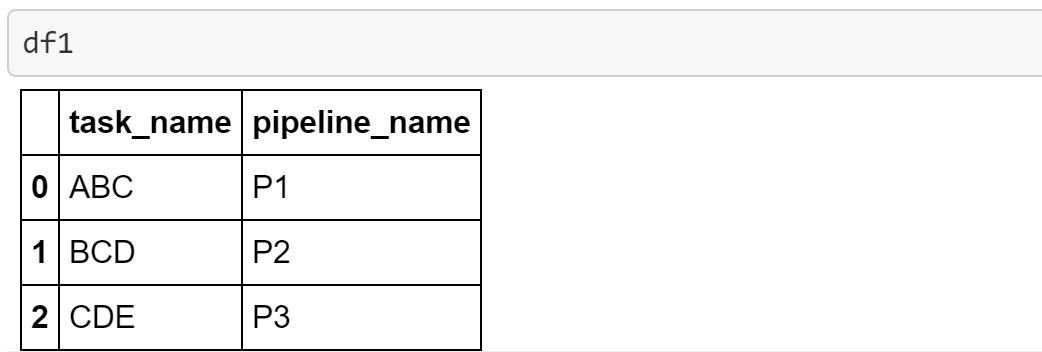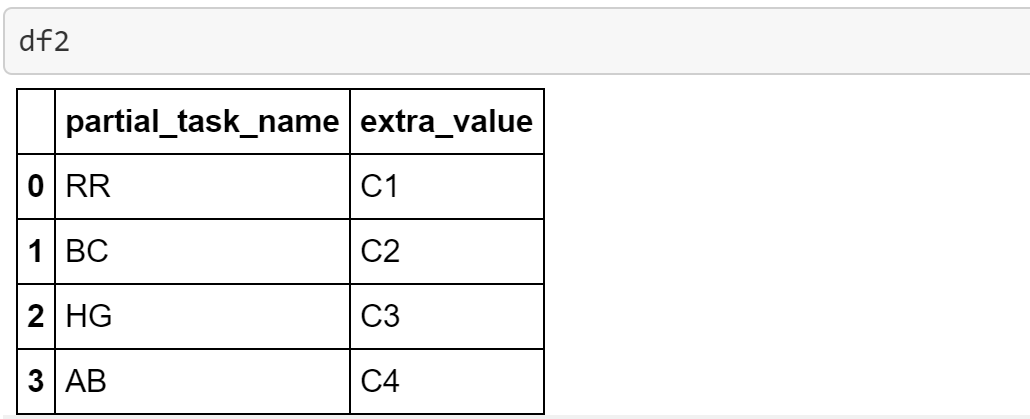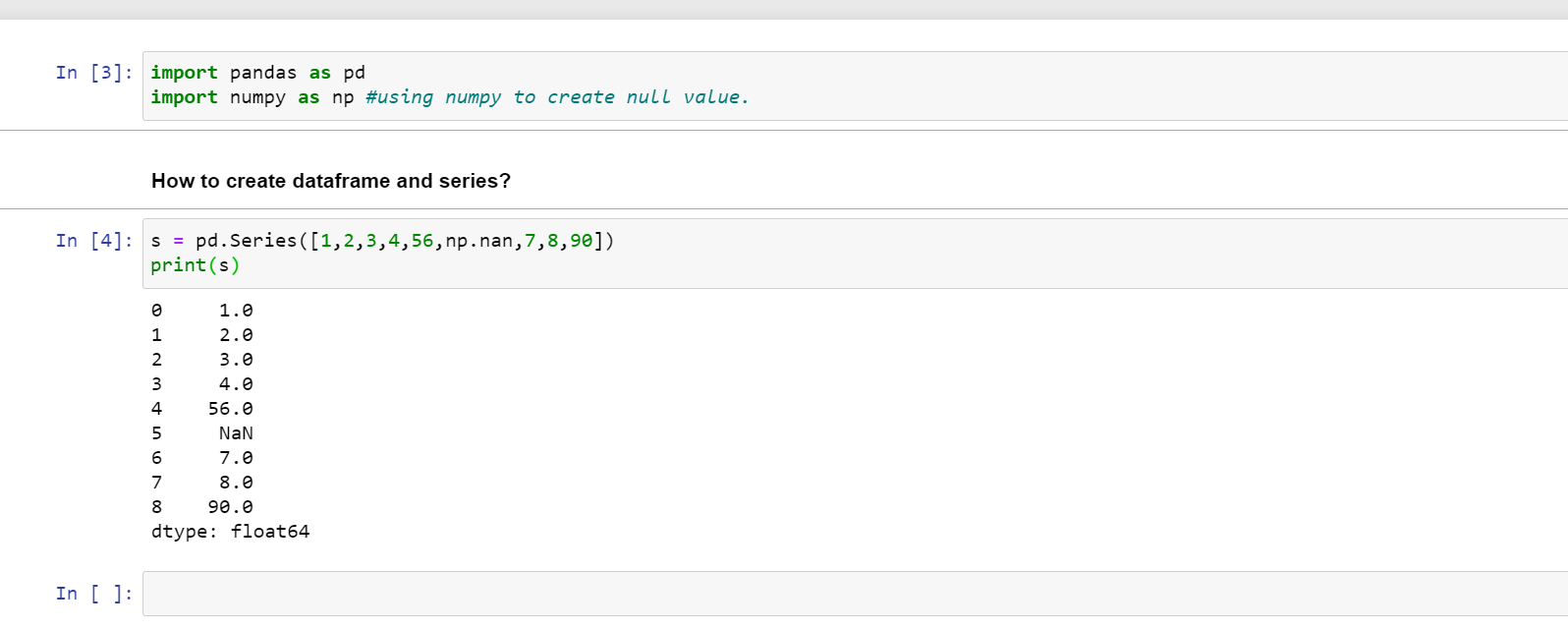1599163620

# Joining DataFrames by substring match with Python Pandas

## The Source Code

The next Python Pandas code made it for Jupyter Notebook is available in GitHub, and It answers the question: “Which tasks don’t match?”

## The Data

The first part of the code creates two DataFrames: **df1 **and df2.The **df1 **DataFrame has the complete name of the tasks in the **task_name **column.And the **df2 **DataFrame has a substring in the **partial_task_name **column.Look that the value **BC **in **partial_task_name is a substring of ABC **and BCD, the expected result must produce many rows for this case, but how can we get many rows? The answer is using a Cartesian Product or Cross Join.

## The Join

To do a Cartesian Product in Pandas, do the following steps:

1. Add a dummy column with the same value en each of the DataFrames
2. Do a join by the new column
3. Remove the new column in each DataFrame
``````df1['join'] = 1
df2['join'] = 1

dfFull = df1.merge(df2, on='join').drop('join', axis=1)
df2.drop('join', axis=1, inplace=True)
``````

## The Match

The next step is to add a new column in the result DataFrame returning if the **partial_task_name **column is in the **task_name **column. We are going to use a lambda and “find” function where the result is ≥ 0

#python #substring-search #cross-join #pandas #cartesian-product

## Buddha Community1623370500

## Practice Problems: How To Join DataFrames in Pandas

Hey - Nick here! This page is a free excerpt from my \$199 course Python for Finance, which is 50% off for the next 50 students.

It’s now time for some practice problems! See below for details on how to proceed.

### Course Repository & Practice Problems

All of the code for this course’s practice problems can be found in this GitHub repository.

There are two options that you can use to complete the practice problems:

• Open them in your browser with a platform called Binder using this link (recommended)
• Download the repository to your local computer and open them in a Jupyter Notebook using Anaconda (a bit more tedious)

Note that binder can take up to a minute to load the repository, so please be patient.

Within that repository, there is a folder called `starter-files` and a folder called `finished-files`. You should open the appropriate practice problems within the `starter-files` folder and only consult the corresponding file in the `finished-files` folder if you get stuck.

The repository is public, which means that you can suggest changes using a pull request later in this course if you’d like.

#dataframes #pandas #practice problems: how to join dataframes in pandas #how to join dataframes in pandas #practice #/pandas/issues.1619510796

## Lambda, Map, Filter functions in python

Welcome to my Blog, In this article, we will learn python lambda function, Map function, and filter function.

Lambda function in python: Lambda is a one line anonymous function and lambda takes any number of arguments but can only have one expression and python lambda syntax is

Syntax: x = lambda arguments : expression

Now i will show you some python lambda function examples:

#python #anonymous function python #filter function in python #lambda #lambda python 3 #map python #python filter #python filter lambda #python lambda #python lambda examples #python map

1586702221

## Python Pandas Objects - Pandas Series and Pandas Dataframe

In this post, we will learn about pandas’ data structures/objects. Pandas provide two type of data structures:-

### Pandas Series

Pandas Series is a one dimensional indexed data, which can hold datatypes like integer, string, boolean, float, python object etc. A Pandas Series can hold only one data type at a time. The axis label of the data is called the index of the series. The labels need not to be unique but must be a hashable type. The index of the series can be integer, string and even time-series data. In general, Pandas Series is nothing but a column of an excel sheet with row index being the index of the series.

### Pandas Dataframe

Pandas dataframe is a primary data structure of pandas. Pandas dataframe is a two-dimensional size mutable array with both flexible row indices and flexible column names. In general, it is just like an excel sheet or SQL table. It can also be seen as a python’s dict-like container for series objects.

#python #python-pandas #pandas-dataframe #pandas-series #pandas-tutorial1623992220

## Deep Dive Into Pandas DataFrame Join — pd.join()

### A deep dive visual tutorial on how to join columns with other data frames in pandas

he` join( )` function of the pandas’ library is used to join columns of another DataFrame. It can efficiently join columns with another DataFrame on index or on a key column. We can also join multiple DataFrame objects by passing a list. Let’s start by understanding its’ syntax and parameters. The companion materials for this tutorial can be found under our resources section.

### Table of Content:

#artificial-intelligence #deep dive into pandas dataframe join — pd.join() #pandas #pandas dataframe #pd.join() #dive1602550800

## Pandas in Python

Pandas is used for data manipulation, analysis and cleaning.

What are Data Frames and Series?

Dataframe is a two dimensional, size mutable, potentially heterogeneous tabular data.

It contains rows and columns, arithmetic operations can be applied on both rows and columns.

Series is a one dimensional label array capable of holding data of any type. It can be integer, float, string, python objects etc. Panda series is nothing but a column in an excel sheet.

### How to create dataframe and series?

s = pd.Series([1,2,3,4,56,np.nan,7,8,90])

print(s)How to create a dataframe by passing a numpy array?

1. d= pd.date_range(‘20200809’,periods=15)
2. print(d)
3. df = pd.DataFrame(np.random.randn(15,4), index= d, columns = [‘A’,’B’,’C’,’D’])
4. print(df)

#pandas-series #pandas #pandas-in-python #pandas-dataframe #python